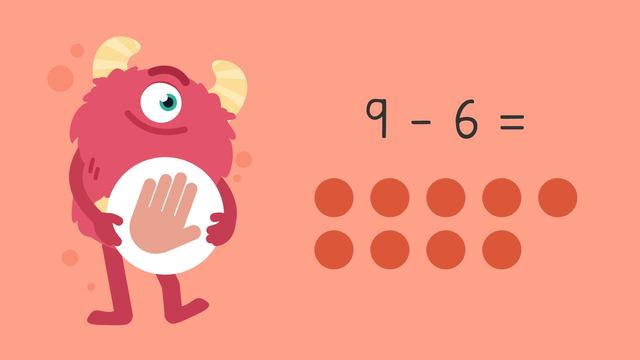# Subtraction Word Problems up to Twenty—Let's Practice!Rating

Ø 4.3 / 3 ratings
The authorsTeam Digital

## Basics on the topicSubtraction Word Problems up to Twenty—Let's Practice!

Today we are solving subtraction word problems with Razzi! This video contains examples to help you further practice and grow confident in this topic.

### TranscriptSubtraction Word Problems up to Twenty—Let's Practice!

Razzi says get these items ready (...) Because today we're going to practice... Subtraction Word Problems up to Twenty. It's time to begin! Write a number sentence and use drawings to solve the word problem. There are eighteen cookies. Kami gives fourteen to her friends. How many cookies are left? Since Kami started with eighteen cookies and gave fourteen away... the number sentence is eighteen minus fourteen equals blank. Now draw eighteen circles, (...) and cross off fourteen. Last, count how many are left. Did you also get four? Let's tackle the next problem! Write a number sentence and use drawings to solve the word problem. Rowan has twelve crayons. They let Xen borrow three. How many crayons does Rowan have now? Since Rowan started with twelve crayons and let Xen borrow three... the number sentence is twelve minus three equals blank. Now draw twelve circles, (...) and cross off three. Last, count how many are left. Did you also get nine? Let's try one more! Write a number sentence and use drawings to solve the word problem. Dorian has nine squishies. Peyton has six squishies. How many more squishies does Dorian have? Since Dorian has nine squishies and Peyton has six... the number sentence is nine minus six equals blank. Now draw nine circles, (...) and cross off six. Last, count how many are left. Did you also get three? Razzi had so much fun practicing with you today! See you next time!

## Subtraction Word Problems up to Twenty—Let's Practice! exercise

Would you like to apply the knowledge you’ve learned? You can review and practice it with the tasks for the video Subtraction Word Problems up to Twenty—Let's Practice!.
• ### How many party hats are left?

Hints

Sam started with 12 hats and 10 were taken so we are subtracting 12 - 10. How can you use the picture to help you.

If we cross 10 hats off, how many are we left with?

Solution

Sam would have 2 hats left over.

12 - 10 = 2

• ### How many toys are left?

Hints

Create a number sentence.

subtract
• How many toys does Zak still have?

The number sentence we are trying to solve is 13 - 7. What could you do to help you solve this?

Try drawing 13 dots and crossing 7 off. How many are left?

Solution

Zak would have 6 toys left.

13 - 7 = 6

• ### Can you solve these problems?

Hints

Look for how many pieces of fruit there was to start with. This is the first number in your number sentence.

How many pieces of fruit were eaten? This is the second number in your number sentence.

Solution

• There are 5 people in the Smith family. In their fruit bowl there were 11 bananas. On Monday, every member of the family took a banana. How many bananas are left? 6
11 - 5 = 6

• Mr. Smith gave his daughter 15 strawberries but she only ate 9. That means there are 6 strawberries left.
15 - 9 = 6

• ### Solve the subtraction problems.

Hints

Figure out what the number sentence you are trying to solve for each problem is.

Subtract the smaller number in the problem from the larger number.

Solution
1. Harper had bought 12 pieces of candy with their pocket money. They gave 6 to their brother. Harper had 6 pieces of candy left. 12 - 6 = 6
2. Daniel's mom gave him 17 green beans at lunch. Daniel ate 15 beans. There were 2 green beans left. 17 - 15 = 2
3. Mo was given 14 dollars for his birthday. He spent 8 of them. Now Mo has 6 dollars left. 14 - 8 = 6
4. Eden has a set of 20 sparkly pens. Sasha has 13. Eden has 7 more pens than Sasha. 20 - 13 = 7
• ### How many cakes does Betty have left?

Hints

Betty started with 8 cakes and sold 5 so we are solving 8 - 5.

You can cross 5 off like this. How many are left?

Solution

Betty has 3 cakes left.

8 - 5 = 3

• ### The Rainbow Bakery.

Hints

What is the number sentence you are trying to solve?

Remember, if you are finding the difference, you still use a subtraction equation.

Subtract the smaller number from the larger number.

Draw dots to represent the larger number then cross off the amount shown by the smaller number. What you have left is your answer.

Solution
• It is a busy day at The Rainbow Bakery. There were 19 loaves of bread this morning but 12 have already sold. Now there are 7 loaves left. 19 - 12 = 7
• The rainbow bagels are also a popular choice today! There were 18 at the start of the day and 15 have sold. Now there are only 3 left! 18 - 15 = 3
• The customers think the cakes look delicious! There were 14 at the start of the day and now 5 are left. That means that 9 have been sold. 14 - 5 = 9
• Sarah bought 12 biscuits and Will bought 8. Sarah bought 4 more biscuits than Will. 12 - 8 = 4
• Just before they close for the day Mrs. Rose popped in and bought 8 of the 15 items that were left. The Rainbow Bakery have finished the day with 7 items left. 15 - 8 = 7